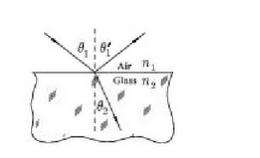# Problem: 1. A light ray is incident on a reflecting surface. If the light ray makes a 40° angle with respect to the normal to the surface, what is the exact angle (in degrees) made by the reflected ray relative to the normal?2. The light ray that makes the angle θ2 is the reflected ray.True or False

###### FREE Expert Solution

1.

For a reflecting surface:

$\overline{){{\mathbf{\theta }}}_{{\mathbf{i}}}{\mathbf{=}}{{\mathbf{\theta }}}_{{\mathbf{r}}}}$

100% (146 ratings)###### Problem Details

1. A light ray is incident on a reflecting surface. If the light ray makes a 40° angle with respect to the normal to the surface, what is the exact angle (in degrees) made by the reflected ray relative to the normal?

2. The light ray that makes the angle θ2 is the reflected ray.

True or False